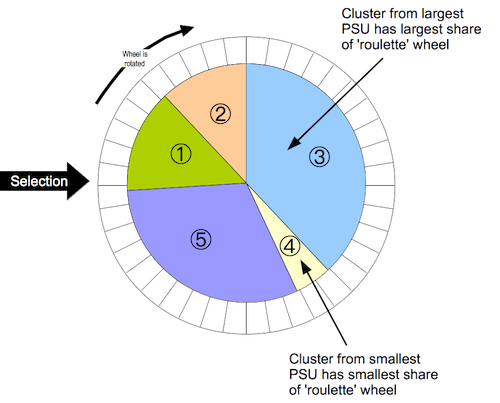# Blocked Weighted Bootstrap

#### 2022-05-30

The blocked weighted bootstrap is an estimation technique for use with data from two-stage cluster sampled surveys in which either prior weighting (e.g. population-proportional sampling or PPS as used in Standardized Monitoring and Assessment of Relief and Transitions or SMART surveys) or posterior weighting (e.g. as used in Rapid Assessment Method or RAM and Simple Spatial Sampling Method or S3M surveys).

The bootstrap technique is described in this article. The blocked weighted bootstrap used in RAM and S3M is a modification to the percentile bootstrap to include blocking and weighing to account for a complex sample design.

With RAM and S3M surveys, the sample is complex in the sense that it is an unweighted cluster sample. Data analysis procedures need to account for the sample design. A blocked weighted bootstrap can be used:

Blocked: The block corresponds to the primary sampling unit ($$PSU = cluster$$). PSUs are resampled with replacement. Observations within the resampled PSUs are also sampled with replacement.

Weighted: RAM and S3M samples do not use population proportional sampling (PPS) to weight the sample prior to data collection (e.g. as is done with SMART surveys). This means that a posterior weighting procedure is required. bbw uses a “roulette wheel” algorithm (see Figure 1 below) to weight (i.e. by population) the selection probability of PSUs in bootstrap replicates.

Figure 1: Roulette wheel algorithmIn the case of prior weighting by PPS all clusters are given the same weight. With posterior weighting (as in RAM or S3M) the weight is the population of each PSU. This procedure is very similar to the fitness proportional selection technique used in evolutionary computing.

A total of $$m$$) PSUs are sampled with replacement for each bootstrap replicate (where $$m$$) is the number of PSUs in the survey sample).

The required statistic is applied to each replicate. The reported estimate consists of the 0.025th (95% LCL), 0.5th (point estimate), and 0.975th (95% UCL) quantiles of the distribution of the statistic across all survey replicates.

Early versions of the bbw did not resample observations within PSUs following:

Cameron AC, Gelbach JB, Miller DL, Bootstrap-based improvements for inference with clustered errors, Review of Economics and Statistics, 2008:90;414–427 https://doi.org/10.1162/rest.90.3.414

and used a large number (e.g. $$3999$$) survey replicates. Current versions of the bbw resample observations within PSUs and use a smaller number of survey replicates (e.g. $$n = 400$$). This is a more computationally efficient approach.

The main reason to use bbw is that the bootstrap allows a wider range statistics to be calculated than model-based techniques without resort to grand assumptions about the sampling distribution of the required statistic. A good example for this is the confidence interval on the difference between two medians which might be used for many socio-economic variables. The bbw also allows for a wider range of hypothesis tests to be used with complex sample survey data.